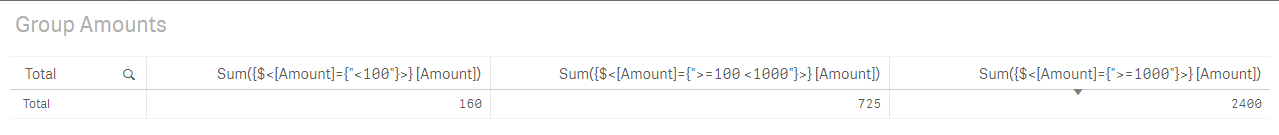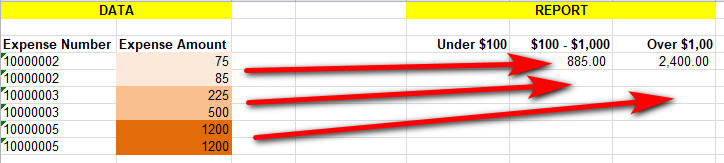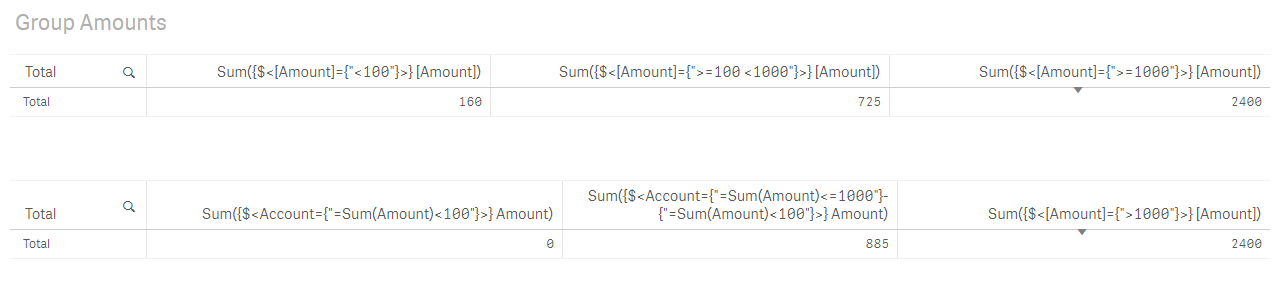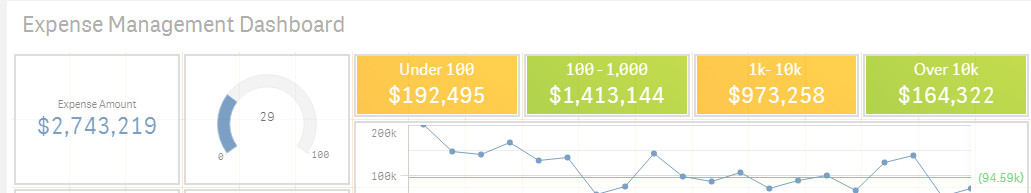Announcements
You can succeed best and quickest by helping others to succeed. Join the conversation.
cancel
Showing results for
Did you mean:Anonymous
Not applicable

## Sum in ranges by a group category

Hi there

I have expense lines loaded that group up into an Expense Reports (1 or more lines)

I am currently showing the total amount in each of these groups using these expressions

• Sum({\$<[Expense Amount]={">=10000"}>} [Expense Amount])
• Sum({\$<[Expense Amount]={">=1000,<=10000"}>} [Expense Amount])
• Sum({\$<[Expense Amount]={">=100,<=999"}>} [Expense Amount])
• Sum({\$<[Expense Amount]={"<100"}>} [Expense Amount])What I need is to do is add the total for each Expense Report in to one of the ranges listed above

Neil

1 Solution

Accepted SolutionsSpecialist

This is the 3rd one: Sum({\$<Account={"=Sum(Amount)>1000"}>} Amount)

9 RepliesSpecialist

Hi Neil,

I'm not getting what you need. Looks like you want Sum([Expense Amount]) but that can't be...

Cheers,

LuisAnonymous
Not applicable
Author

sorry for the confusion Luis, this is what I'm trying to achieveHope this helps.Specialist

Like this?Anonymous
Not applicable
Author

I'm so sorry i led you astray.

I've updated the previous image and added it below as well so you can see there are 3 expenses and each has 2 lines.

Expense 10000002 has a total of \$160 and so it should be added into the 100 - 1000 grouping.

Expense 10000003 has a total of \$725 and it should also be added into the 100 - 1000 grouping.

Expense 10000005 has a total of \$2400 and it should be added into the Over 1000 grouping.

So we need to sum up all the Expense Lines for an Expense Number before we decide which grouping to add it to.NeilSpecialist

Ok, check with this one although there must be better ways to write it... I'm just starting with Set Analysis...This is what I'm using to learn Set Analysis: https://community.qlik.com/docs/DOC-4951

I hope this helps

Cheers,

LuisSpecialist

This is the 3rd one: Sum({\$<Account={"=Sum(Amount)>1000"}>} Amount)Anonymous
Not applicable
Author

Hey I think that worked. I got a number. I need to check the number but I have a meeting right now so will check a little later

Thanks so much

NeilSpecialist

no worries mate!Anonymous
Not applicable
Author

You are a legend Luis. That worked perfectly.

I have attached my final expressions below just in case someone else can be helped by this.

Expenses Under \$100

=Sum({\$<[Expense Amount]={"=Sum([Expense Amount])<100"}>} [Expense Amount])

Expense \$100 - \$1,000

=Sum({\$<[Expense Amount]={"=Sum([Expense Amount])<=1000"} - {"=Sum([Expense Amount])<100"} >} [Expense Amount])

Expenses \$1,000 - \$10,000

=Sum({\$<[Expense Amount]={"=Sum([Expense Amount])<=10000"} - {"=Sum([Expense Amount])<1000"} >} [Expense Amount])

Expenses Over \$10,000

=Sum({\$<[Expense Amount]={"=Sum([Expense Amount])>10000"}>} [Expense Amount])Tags
Community Browser